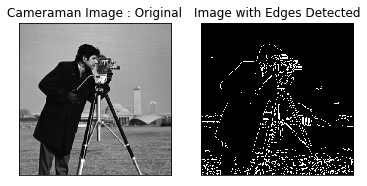# Image Processing Series #3 : Edge Detection in Python Using OpenCV¶Edge Detection means to find out the area or part of an image where the color, brightness, contrast or shades changes by a greater amount or changes sharply. Another way to define edge as the area of image with discontinuities. The output of edge detection is an another image with a line like color shade in the area where there are sharp changes and discontinuities. These line like color shades are typically called edges.

Edge detection is the most commonly technique used in the field of Machine Learning for the purpose of feature engineering.

In [ ]:
```To understand edge detection in more detail consider the following one dimensional whose edge needs to be find out :
[3,10,1,21,23,178,198,205,165,195]

Consider these values to be a part of 2 dimensional image where these values represents the pixel value.

In the above array the value after 4th index or 4th pixel the value changes sharply. Due to big change in pixel value there will be a
huge change in either color shading or brightness or surface orientation of objects in image or material properties or
scene illumination in image etc.

Thus, edges gives very useful information of objects, scenes, surface orientation , brightness material properties of an image.
```
In [ ]:
```There are number of edge detection algorithms. In this blog we will take in consider canny edge detection algorithm.
This is most popular and commonly used edge detection algorithm.

Ia a python code first step is to read the image file in to a variable.
```
In :
```import cv2
import numpy as np
from matplotlib import pyplot as plt

```

Now we will apply Canny function of cv2 which takes three mandatory arguments :

(1) Variable in which image matrix is stored.

(2) Minimum Value

(3) Maximum Value

When the edge pixel value is greater than the Maximum value, then it is marked as a strong edge pixel.
If an edge pixel value is lower than the Maximum value and greater than Minimum value, then it is marked as a weak edge pixel. If the pixel value of edge is lower than the Minimum value, it will be suppressed

In :
```detected_edge_edge = cv2.Canny(cameraman_image,100,200)
```

In the below code we will view both original image and output image with edges detected.
subplot function is used to adjust both original image and output image in to a single view or window of view.

In :
```plt.subplot(121),plt.imshow(cameraman_image,cmap = 'gray')
plt.title('Cameraman Image : Original'), plt.xticks([]), plt.yticks([])
plt.subplot(122),plt.imshow(detected_edge_edge,cmap = 'gray')
plt.title('Image with Edges Detected'), plt.xticks([]), plt.yticks([])
plt.show()
```Few Applications of Edge Detection :

(1) Finger Print Recognition

(2) Object Movement Detection in Video Analysis

(3) Satellite Image Analysis

(4) Object Recognition

(5) Outside Object Distance measurement in Automatic Driving Vehicle

Comment if you would like to have step by step python code for any one of the application above or email at info@storejockey.com